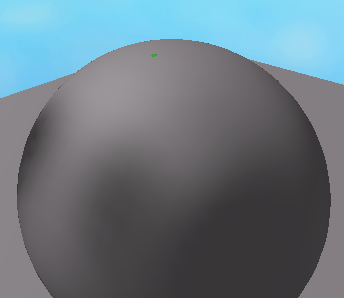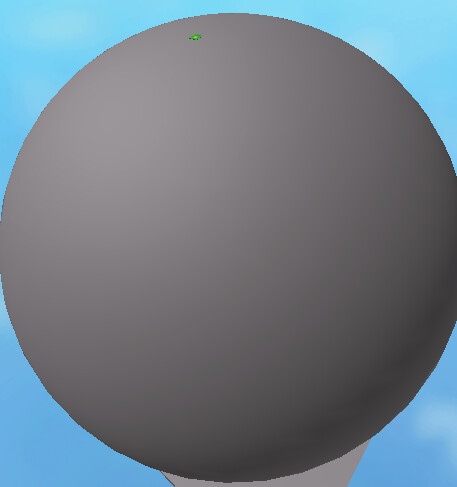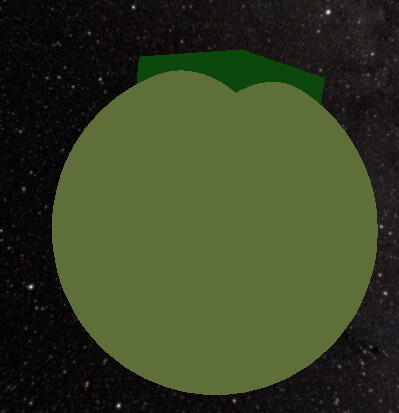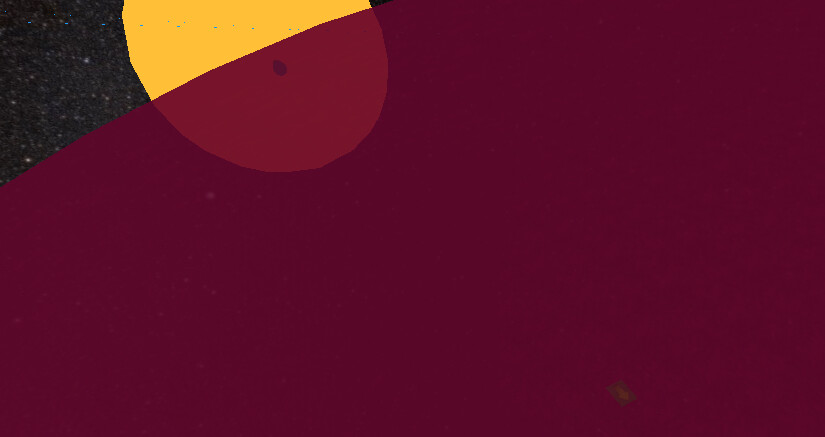# Help on size changing script(Each planet above has a green spawn)
These pictures I sent above varies in different size. Basically, I want to change the size of the model in-game, but the only problem is, I don’t know how to make the other parts, such as that green or the spawn, to not be scaled too high, and in the correct position.Script:

``````local function ScaleModel(model, scale)

for _,v in pairs(model:GetDescendants()) do
if v:IsA("BasePart") then
v.Size = Vector3.new(v.Size.X + scale,v.Size.Y + scale,v.Size.Z + scale)
end
end
end
``````

halp plez

I’m confused what exactly you mean… Do you want to scale all of the parts of the model, besides the green spawn? If so, that’s very easy. Just use an “and” statement in your if statement to check if the name of the part doesn’t match the name of the spawn.

``````if v:IsA("BasePart") and v.Name ~= "SpawnNameHere" then
v.Size = Vector3.new(v.Size.X + scale,v.Size.Y + scale,v.Size.Z + scale)
end``````

I mean, don’t you see the difference of the diagram? The spawn is too big and not in the correct size! The spawn is scaled as unexpected.

1 Like

I believe this post would solve your issue.

1 Like

Just call this function:

``````local function ScaleModel(model, scale)

local primary = model.PrimaryPart
local primaryCf = primary.CFrame

for _,v in pairs(model:GetDescendants()) do
if (v:IsA("BasePart")) then
v.Size = (v.Size * scale)
if (v ~= primary) then
v.CFrame = (primaryCf + (primaryCf:inverse() * v.Position * scale))
end
end
end

return model

end

local planet1 = --
ScaleModel(planet1, 3) --will scale planet1 3 times as large``````
2 Likes

They both works, but now another problem I have encountered is that the parts of the planet when resizing it are not in the correct position. (I use weldconstraints, and it is needed because I have which changes the planets position to add into an orbit)Weld constraints would require a more complicated solution, you might want to destroy them and then re-build them onto the models if possible.

When the cframe property of a part connected to other parts with a weldconstraint is changed, the parts connected to it are moved too. However, if the position property of that part is changed instead, only that spesific part will move. The rotation of the parts isn’t changed in this situation, so setting the position should be an easy fix.

How can you set the position? (Diagram 1, and 2 as the gray planet, is an example of what I wanted) I want it to be scale accurately and exactly looks like the same when scale has changed.

If you’re using the code @XdJackyboiiXd21 posted, replace

``````v.CFrame = (primaryCf + (primaryCf:inverse() * v.Position * scale))
``````

with

``v.Position = primaryCf*(primaryCf:PointToObjectSpace(v.Position)*scale)``

Thanks, but one more help I want is, How can I change the size of the planets by addiction. For an example, the planet has 1k studs in size and I want to change the size by 100 to 1048, as random, how can I possibly do that?

If I understood correctly, this should help.

``````local function getScaleForResizing(currentSize, sizeToAdd)
end``````

How can I apply that script to the code XdJackyboiiXd21 sent, because I tried to apply it but I don’t know how to apply it.

``````local planet = -- the planet model

local function ScaleModel(model, scale)

local primary = model.PrimaryPart
local primaryCf = primary.CFrame

for _,v in pairs(model:GetDescendants()) do
if (v:IsA("BasePart")) then
v.Size = (v.Size * scale)
if (v ~= primary) then
v.Position = primaryCf*(primaryCf:PointToObjectSpace(v.Position)*scale)
end
end
end

return model

end

local size = planet.PrimaryPart.Size.X -- just an example
local sizeToAdd = 100 -- just an example